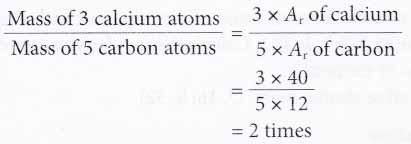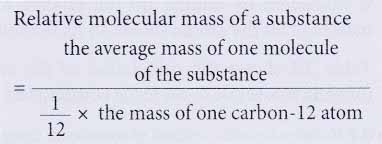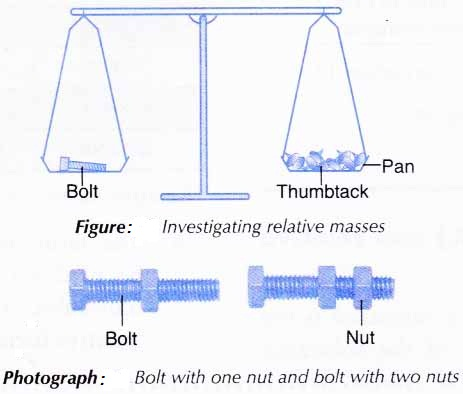# What is the Relative Atomic Mass and Relative Molecular Mass of an Element?

## Relative Atomic Mass Formula

The earlier development of relative atomic mass

1. An atom is very tiny. Therefore, it is impossible to determine its mass by weighing. So, chemists compare the mass of an atom with a standard atom.
2. The mass of an atom when compared to a standard atom is known as its relative atomic mass (Ar). It has no unit.
3. At first, chemists use the hydrogen atom as the standard atom because it is the lightest. The mass of one hydrogen atom was assigned 1 unit.
4. For example, a helium atom is 4 times heavier than a hydrogen atom and thus the relative atomic mass of helium is 4.
5. However, many elements cannot react with hydrogen. Thus, the relative masses of these elements cannot be determined experimentally. Furthermore, the existence of hydrogen as a gas at room temperature makes it difficult to handle.
6. So, the oxygen atom replaced hydrogen as the standard atom.
7. However, problems arose when the existence of three isotopes of oxygen were discovered. Chemists used natural oxygen containing all the three isotopes as the standard whereas physicists used the isotope oxygen-16 as the standard.

## Relative atomic mass (Ar) based on carbon-12

Finally in 1961, a new single standard, the carbon-12 atom was agreed upon internationally.

1. The mass of one carbon-12 atom was assigned exactly 12 units.
2. Carbon-12 was chosen for a few reasons.
(a) Carbon-12 was already being used as the reference standard in mass spectrometers.
(b) Many elements can combine with carbon -12.
(c) Carbon-12 exists as a solid at room temperature and thus can be handled easily.
(d) Carbon-12 is the most abundant carbon isotope, occurring about 98.89%. Thus, the mass of exactly 12 units assigned to one carbon-12 atom is an accurate value.
3. Based on the carbon-12 scale, the relative atomic mass (Ar) of an element is defined as the average mass of one atom of the element when compared with one twelfth of the mass of a carbon-12 atom.4. For example, the average mass of one sodium atom is 23 times more than the mass of one twelfth of a carbon-12 atom. Therefore, the relative atomic mass of sodium is 23.
5. The present relative atomic masses of elements are based on the carbon-12 scale and can be found in the Periodic Table of Elements.

Note:

1. Why is the relative atomic mass of an element has no unit?
Relative atomic mass is not the actual mass of an atom. It is only a comparison value. Therefore, relative atomic mass has no unit.
2. The relative atomic masses for most elements are integers. However, the relative atomic mass of chlorine is taken as 35.5 and is not an integer. Why?
Note that relative atomic mass is determined based on the average mass of elements. For most elements, only one of their isotopes occurs in great abundance. Hence, their relative atomic masses are very close to integers and therefore can be rounded up. Natural chlorine consists of two isotopes in significant amounts — 75% chlorine-35 and 25% chlorine-37. Therefore, the relative atomic mass works out to be 35.5 and thus cannot be rounded off.

## Relative Atomic Mass Problems with Solutions

1. Calculate how many times heavier are 3 calcium atoms compared to 5 carbon atoms.
[Relative atomic mass: C, 12; Ca, 40]
Solution:2. How many magnesium atoms will have the same mass as two silver atoms?
[Relative atomic mass: Mg, 24; Ag, 108]
Solution:## Relative molecular mass (Mr) and Relative formula mass (Fr)

1. Relative molecular mass of a substance is the average mass of a molecule of the substance when compared with one twelfth of the mass of one carbon-12 atom.2. For example, the relative molecular mass of water is 18. This means that the average mass of one molecule of water is 18 times more than the mass of one twelfth of a carbon-12 atom.
3. A molecule is made up of a number of atoms. Therefore, the relative molecular mass of a substance is calculated by adding up the relative atomic masses of all the atoms present in a molecule of the substance.
4. For this reason, it is essential to know the molecular formula of the substance first.
5. Table below shows how to calculate the relative molecular masses of some substances.
 Substance Molecular formula Relative molecular mass, Mr Hydrogen gas H2 2(1) = 2 Oxygen gas O2 2(16) = 32 Water H2O 2(1) + 16 = 18 Propane C3H8 3(12) + 8(1) = 44 Ethanol C2H5OH 2(12) + 5(1) + 16 + 1 = 46

Table: Relative molecular masses of some substances
[Relative atomic mass: H, 1; C, 12; O, 16]

6. The term ‘relative molecular mass’ can only be used for substances that are made up of molecules. For ionic compounds, the term ‘relative formula mass’ or Fr is used instead.
7. Similar to the relative molecular mass, the relative formula mass of an ionic compound is calculated by adding up the relative atomic masses of all the atoms shown in its formula.
8. Table below shows the calculation of the relative formula masses of some ionic compounds.
Table: Relative formula masses of some ionic compounds

 Substance Molecular formula Relative formula mass, Fr Sodium chloride NaCl 23 + 35.5 = 58.5 Potassium oxide K2O 2(39) + 16 = 94 Copper(ll) chloride CuCl2 64 + 2(35.5)= 135 Zinc nitrate Zn(NO3)2 65+ 2[14 +3(16)] = 189 Aluminium sulphate AI2(SO4)3 2(27)+ 3[32 +4(16)] = 342 Hydrated copper(ll) sulphate CuSO4.5H2O 64 + 32 + 4(16) + 5[2(1) + 16] = 250

Relative atomic mass: H, 1; N, 14; O, 16; Na, 23; Al, 27; S, 32; Cl, 35.5; K, 39; Cu, 64; Zn, 65]

9. If we know the relative molecular or formula mass of a compound, we can find out the relative atomic mass of any unknown element in the compound.

## Relative molecular mass and Relative formula mass Problems with Solutions

1. Calculate the relative molecular mass of ammonia.
[Relative atomic mass: H, 1; N, 14]
Solution:
Molecular formula of ammonia is NH3.
Relative molecular mass of ammonia
= Ar of nitrogen + (3 x Ar of hydrogen)
= 14+3(1)
=17

2. The relative formula mass of a compound with the formula Y2SO4 is 142. Calculate the relative atomic mass of element Y.
[Relative atomic mass: O, 16; S, 32]
Solution:
Let the relative atomic mass of element Y = y
Given that the relative formula mass of Y2SO4 = 142
Therefore, 2y + 32 + 4(16) = 142
2y + 96 =142
2y = 46
y = 46/2
= 23
So, the relative atomic mass of element Y is 23.

## Determination of relative atomic mass and relative molecular mass of an element

Aim: To investigate the concepts of relative atomic mass and relative molecular mass using analogy.
Materials: 5 cm bolts, nuts and thumbtacks.
Apparatus: Twin-pan balance.
Procedure:
1. A bolt is placed on one pan of the balance as shown in Figure below.
2. Enough thumbtacks are placed on the other pan to balance the two pans.
3. The number of thumbtacks used is counted and recorded.
4. Steps 1 to 3 are repeated using a nut, a bolt with a nut, and a bolt with two nuts, one at a time, in place of the bolt.Results:

 Object Number of thumbtacks used Relative mass of object (compared to a thumbtack) Bolt 20 20 Nut 5 5 Bolt with a nut 25 25 Bolt with two nuts 30 30

Discussion:

1. In this activity,
(a) thumbtack, bolt and nut represent the atoms of three elements.
(b) thumbtack is taken as the standard for the comparison of mass. The mass of one thumbtack is assigned 1 unit.
(c) bolt with a nut and bolt with two nuts represent two molecules made up of the ‘elements’ bolt and nut.
2. Since the mass of one bolt is equivalent to the mass of 20 thumbtacks, the mass of one bolt is 20 units. Therefore,3. In the same way, the relative atomic mass of the nut is 5.
4. It is found that:
(a) relative molecular mass of bolt with one nut
= relative atomic mass of bolt + relative atomic mass of nut = 20 + 5 = 25
(b) relative molecular mass of bolt with two nuts
= relative atomic mass of bolt + (2 x relative atomic mass of nut)
= 20 + 2(5)
= 30
5. Similarly, the relative molecular mass of a substance is equivalent to the sum of the relative atomic masses of all the atoms that make up one molecule of the substance.
6. It is proven in this activity that we do not need to know the actual mass of atoms or molecules to determine their relative masses.

Conclusion:
Relative atomic or molecular mass can be determined by comparing the mass of one atom or molecule with the mass of a standard atom respectively.